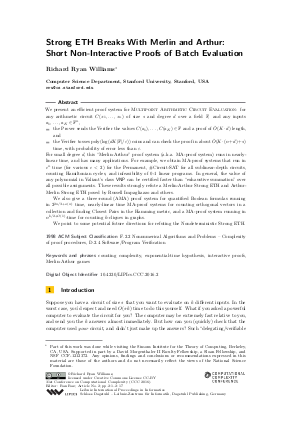Document# Strong ETH Breaks With Merlin and Arthur: Short Non-Interactive Proofs of Batch Evaluation

### Author Richard Ryan Williams## File

LIPIcs.CCC.2016.2.pdf
• Filesize: 0.52 MB
• 17 pages

## Cite As

Richard Ryan Williams. Strong ETH Breaks With Merlin and Arthur: Short Non-Interactive Proofs of Batch Evaluation. In 31st Conference on Computational Complexity (CCC 2016). Leibniz International Proceedings in Informatics (LIPIcs), Volume 50, pp. 2:1-2:17, Schloss Dagstuhl - Leibniz-Zentrum für Informatik (2016)
https://doi.org/10.4230/LIPIcs.CCC.2016.2

## Abstract

We present an efficient proof system for Multipoint Arithmetic Circuit Evaluation: for every arithmetic circuit C(x_1,...,x_n) of size s and degree d over a field F, and any inputs a_1,...,a_K in F}^n, - the Prover sends the Verifier the values C(a_1), ..., C(a_K) in F and a proof of ~O(K * d) length, and - the Verifier tosses poly(log(dK|F|epsilon)) coins and can check the proof in about ~O}(K * (n + d) + s) time, with probability of error less than epsilon. For small degree d, this "Merlin-Arthur" proof system (a.k.a. MA-proof system) runs in nearly-linear time, and has many applications. For example, we obtain MA-proof systems that run in c^{n} time (for various c < 2) for the Permanent, #Circuit-SAT for all sublinear-depth circuits, counting Hamiltonian cycles, and infeasibility of 0-1 linear programs. In general, the value of any polynomial in Valiant's class VP can be certified faster than "exhaustive summation" over all possible assignments. These results strongly refute a Merlin-Arthur Strong ETH and Arthur-Merlin Strong ETH posed by Russell Impagliazzo and others. We also give a three-round (AMA) proof system for quantified Boolean formulas running in 2^{2n/3+o(n)} time, nearly-linear time MA-proof systems for counting orthogonal vectors in a collection and finding Closest Pairs in the Hamming metric, and a MA-proof system running in n^{k/2+O(1)}-time for counting k-cliques in graphs. We point to some potential future directions for refuting the Nondeterministic Strong ETH.
##### Keywords
• counting complexity
• exponential-time hypothesis
• interactive proofs
• Merlin-Arthur games

## Metrics

• Access Statistics
• Total Accesses (updated on a weekly basis)
0